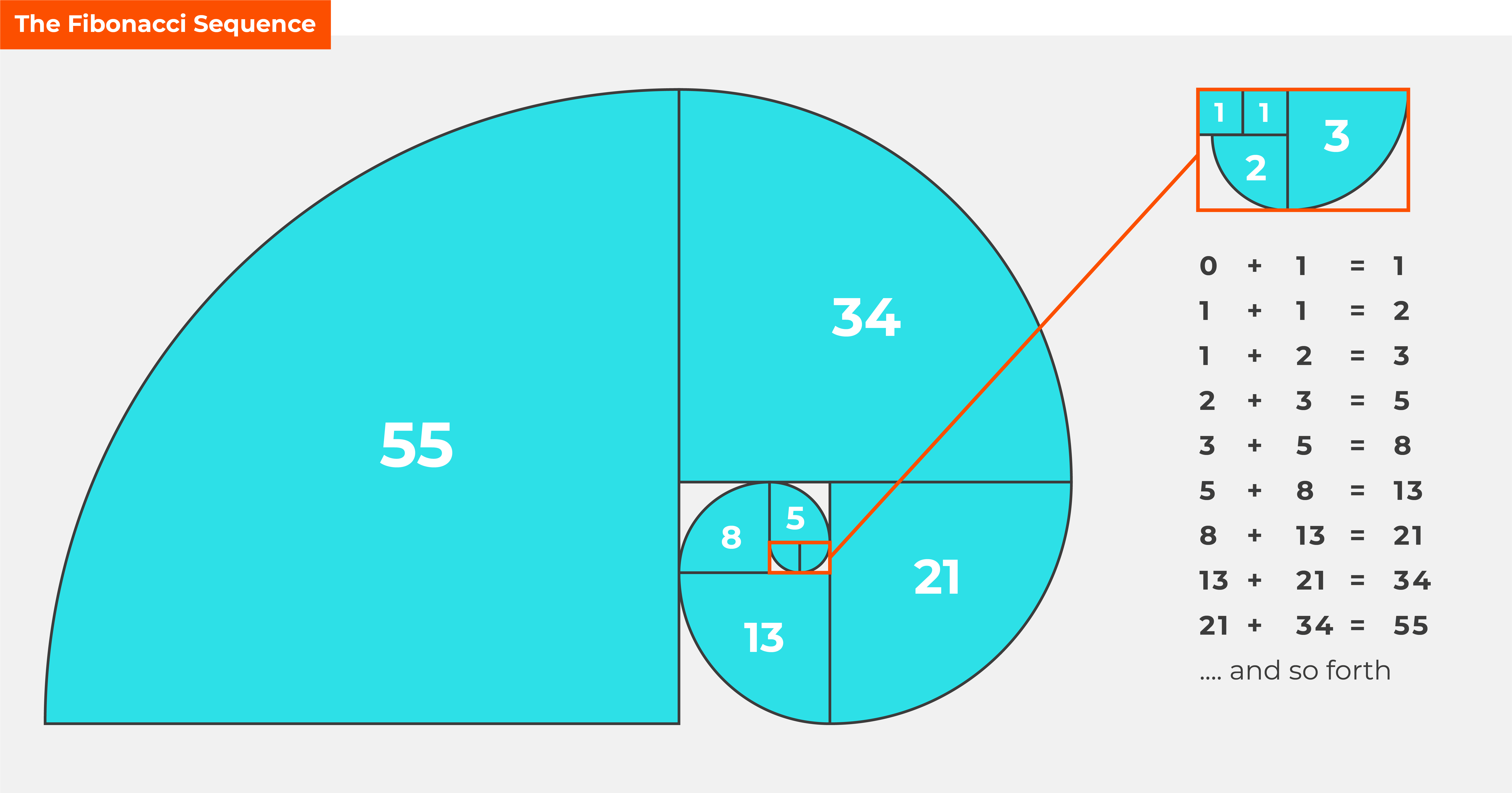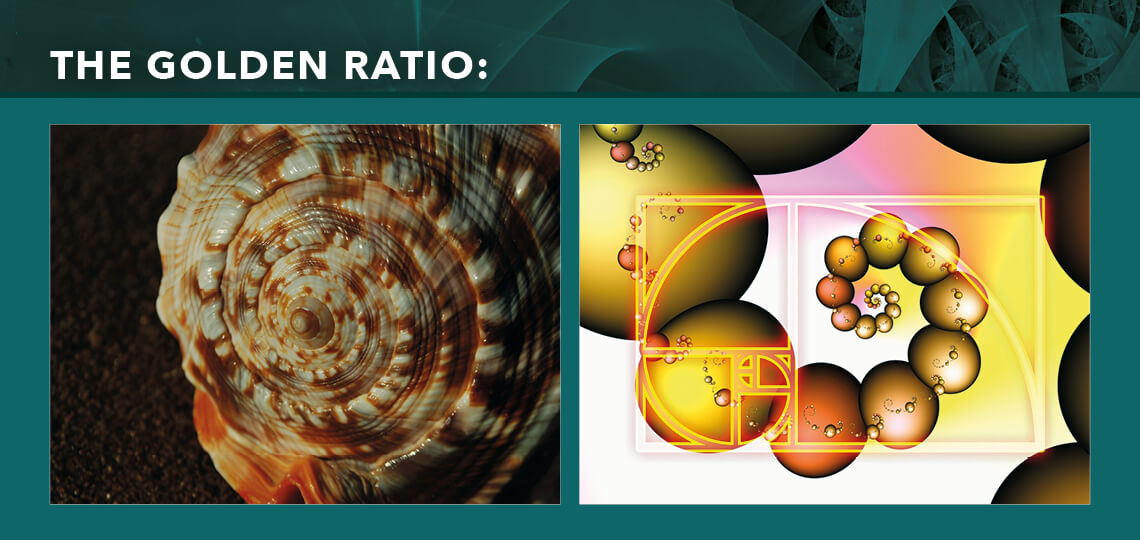Scroll Down

# What is the Fibonacci Sequence?

You may have heard of the Fibonacci Sequence from your days studying Maths, or maybe even art – but did you know that this ancient technique is also used in trading?

The Fibonacci sequence was named after the Italian mathematician, Leonardo of Pisa (later known as Fibonacci). He wrote about the sequence in his book, Liber Abaci, all the way back in 1202, and introduced the Hindu/Arabic numerical system to the Western world.

The Fibonacci sequence, commonly represented as Fn, is the sum of the preceding two numbers in the sequence starting at zero.

Below shows the sequence:## The Golden Ratio

Although commonly used in Maths, the Fibonacci Sequence is also talked about in the world of art and nature. It comes down to aesthetics and creating a sense of beauty through harmony and proportion in the shape of spirals.

In finance, analysts use the Golden Ratio to look at repetitive trends to determine support and resistance levels. Each number in the sequence is approximately 1.618 times greater than the previous number:

144 divided by 89 = 1.617977

55 divided by 34 = 1.617647

The key Fibonacci Ratio of 61.8% is found by dividing any number within the ratio by the next number in the sequence:

If we divide 89 by 144 we get 0.61805

If we divide 34 by 55 we get 0.61818## Fibonacci retracement levels

In trading, Fibonacci retracement levels are used to find out the critical points of an asset. The most common retracement levels are 61.8%, 38.2% and 23.6%.

These levels are found by dividing any number within the sequence with further number counts to the right:

34 divided by 55 = 0.61818

34 divided by 89 = 0.38202

34 divided by 144 = 0.23611

### Fibonacci extension levels

Extension levels are used when an asset extends more than 100% of its previous move. As a trader, you’ll find that the most common extension levels are 161.8%, 261.8% and 423.6%.

These levels are found by dividing any number within the sequence with further number counts to the left:

34 divided by 21 = 1.61904

34 divided by 13 = 2.61538

34 divided by 8 = 4.2500

When you’re trading, you may use the Fibonacci sequence to predict market action in a more agile way. It formulates a trading plan and also determines the risk and reward of the trade. This is especially relevant when combined with Elliott Wave Analysis.